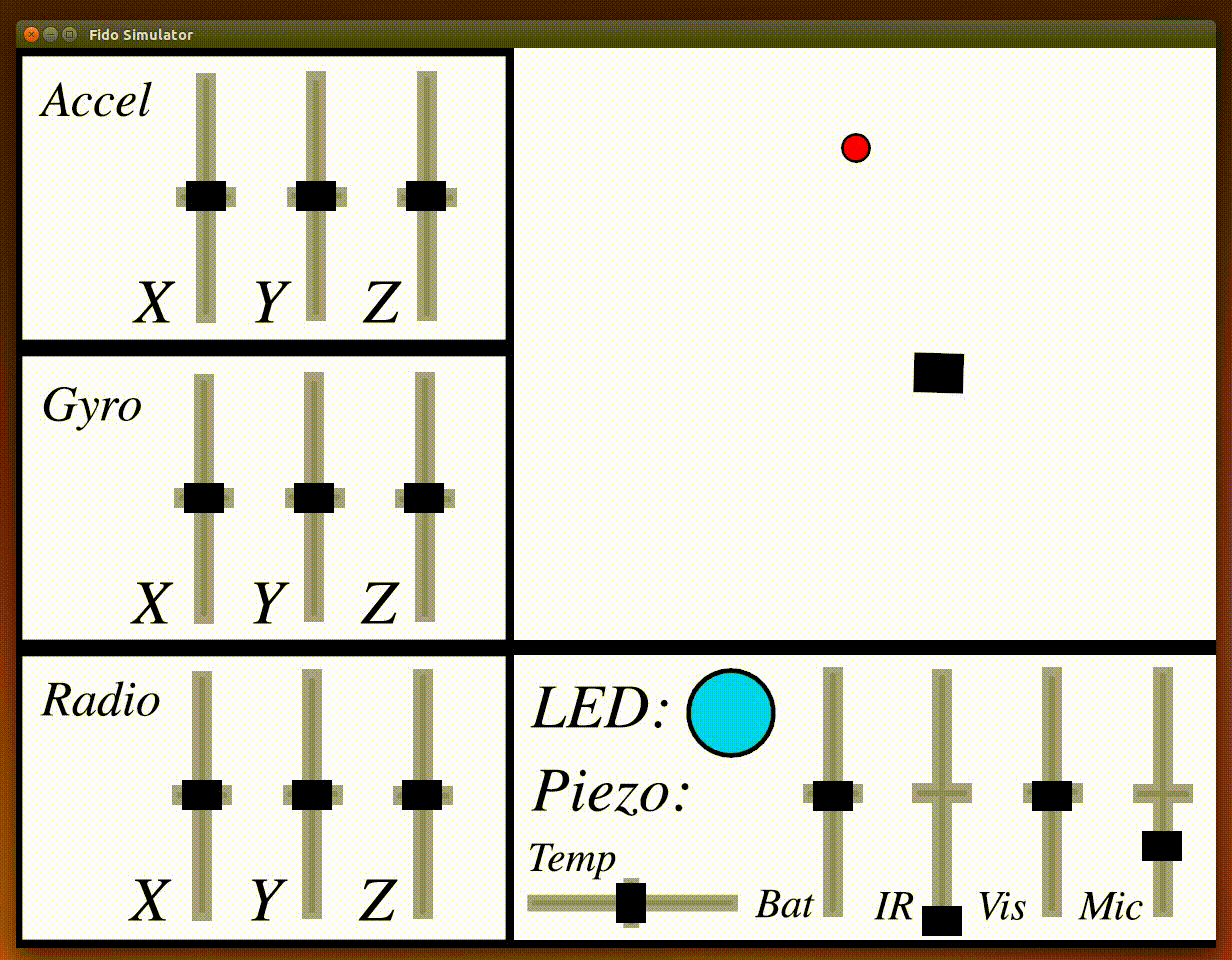## The Fido Project

An open source C++ machine learning library targeted towards embedded electronics and robotics.

Fido is a light-weight, open-source, and highly modular C++ machine learning library. The library is targeted towards embedded electronics and robotics. Fido includes implementations of trainable neural networks, reinforcement learning methods, genetic algorithms, and a full-fledged robotic simulator. Fido also comes packaged with a human-trainable robot control system as described in Truell and Gruenstein.

#### getting started

Download and unzip the latest release. Navigate to the Fido directory and run:

`\$ sudo make install`

And you're done! To use Fido, just include `Fido/Fido.h` in your C++ code and link `/usr/local/lib/fido.a`. For more information please visit the docs.

#### quick examples

##### neural networks

Here is a quick example of training a neural network using backpropagation. We will train the network to perform linear regression.

``````net::NeuralNet neuralNetwork = net::NeuralNet(1, 1, 2, 4, "sigmoid");
neuralNetwork.setOutputActivationFunction("simpleLinear");

std::vector< std::vector<double> > input = { {1}, {2}, {5}, {6} };
std::vector< std::vector<double> > correctOutput = { {2}, {4}, {10}, {12} };

net::Backpropagation backprop = net::Backpropagation();
backprop.train(&neuralNetwork, input, correctOutput);
``````
##### genetic algorithms

Here is a quick example of generating neural networks using genetic algorithms. We will train the network to perform linear regression.

``````#include "Fido/GeneticAlgo.h"
#include <math.h>

std::vector<double> getPopulationFitness(const std::vector<net::NeuralNet> &population) {
std::vector<double> fitnesses;

for(net::NeuralNet network : population) {
double totalError = 0;
for(double input = 1; input < 4; input++) {
totalError += pow(input*2 - network.getOutput({input}), 2);
}
fitnesses.push_back(1/totalError);
}

return fitnesses;
}

int main() {
srand(time(NULL));
gen::GeneticAlgo geneticAlgo = gen::GeneticAlgo(20, 0.4, 0.5, 10, getPopulationFitness);
net::NeuralNet modelNetwork = net::NeuralNet(1, 1, 1, 4, "simpleLinear");
net::NeuralNet bestNetwork = geneticAlgo.getBestNeuralNetwork(600, modelNetwork);
}

``````
##### simulator

Move a robot in a circle in Fido's robotic simulator.``````Simlink simulator;
while(true) {
simulator.setMotors(10, 5, 10, 10);
}``````

While the simulator is not in the most recent release (as it is currently undocumented), it can be found for experimentation on the simulator branch.

##### reinforcement learning

Here is a quick example of using the Fido library to train a robot in our simulator to perform line following using reinforcement learning.

``````rl::FidoControlSystem learner = rl::FidoControlSystem(1, 2, {-1, -1}, {1, 1}, 3);
int goodIterations = 0;

while(goodIterations < 5) {
rl::Action action = learner.chooseBoltzmanAction({ simulator.isLeftOfLine() }, 0.2);

double originalDistance = simulator.distanceFromLine();

sf::Vector2f displacement =  sf::Vector2f(action, action);
sf::Vector2f newPosition = simulator.robot.getPosition() + displacement;
simulator.robot.setPosition(newPosition);

double newDistance = simulator.distanceFromLine();
double rewardValue = (fabs(originalDistance) - fabs(newDistance - originalDistance)) / sqrt(2);
learner.applyReinforcementToLastAction(rewardValue, { simulator.isLeftOfLine() });

if(simulator.distanceFromLine() < 80) goodIterations++;
else goodIterations = 0;
}``````

#### who is fido

Fido was created by programmers Joshua Gruenstein and Michael Truell from the Horace Mann School. The project was started as a universal robot control system using limited feedback, which can be read about here. Fido still continues to be developed in this direction. Fido is open source licensed under the MIT License.